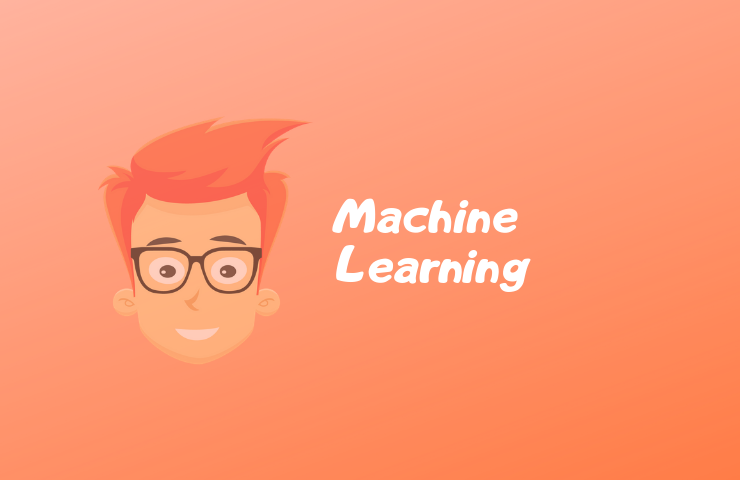# 4 Different Types of Machine Learning Algorithms with ExamplesDo you want to learn more about Machine Learning (ML)? What is Machine Learning? What are the different types of Machine Learning algorithms?

### Introduction to ML

Machine Learning has been a new hype in the field of computer science for a year along with other trending topics such as the Internet of Things, Artificial Intelligence, Big Data

But what is Machine Learning for beginners?

Machine Learning is developing a computer program that improves automatically with experience.

This is a general definition according to me so that it is easily understood by freshers.

By the field of usage and kind of data we are using as input, we can modify this definition accordingly.

For example, Genetic programming is the field of Machine Learning where you essentially evolve a program to complete a task while Neural networks modify their parameters automatically in response to prepared stimuli and expected a response.

Machine Learning can be divided into two following categories based on the type of data we are using as input:

### Types of Machine Learning Algorithms

There are two main types of machine learning algorithms.

• Supervised learning – It is a task of inferring a function from labeled training data.
• Unsupervised learning – It is the task of inferring from a data set having input data without labeled response.

What is a Supervised Machine Learning Algorithm?

It is a task of inferring a function from labeled training data.

What is an Unsupervised Machine Learning Algorithm?

It is the task of inferring from a data set having input data without labeled response.

Both algorithms are further classified into subtypes.

#### Supervised Machine Learning

Supervised learning is where you use an algorithm to learn a mapping function from input to output.

```Y = f(x) where,
x – input variable
Y – output variable
```

The goal is to approximate the mapping function so well that when you have new input data that you can predict the output variable for that data.

Supervised learning problems can be further divided into regression and classification problems.

1. Classification:

Classification is when we have discrete-valued output.

For example,

• When you receive an email, it falls under the “spam” or “inbox” folder. This means email is classified into two types- “spam” or “inbox”.
• Based on the color of the ball, it can be classified into “red”, “blue”, “green” and “all other colors”.

2. Regression:

A regression is when we have continuous-valued output.

For example,

• The price of the house each year.
• Increment in the height of a person over the age.

Algorithm Examples:

Some example of supervised learning algorithms are:

• Linear regression example for regression problems.
• Logistic regression for classification problems.
• Random forest for classification and regression problems.

#### Unsupervised Machine Learning

Unsupervised learning is where you only have input data and there is no corresponding output.

The goal of unsupervised learning is to recognize structure in the data in order to learn more about data.

Unsupervised learning problems can be further divided into clustering and association problems.

3. Clustering:

A clustering problem is where you want to recognize the inherent grouping in the data.

For example,

• grouping customers by who tends to eat veg food items in a restaurant

4. Association:

An association rule learning problem is where you want to discover rules that describe your data.

For example,

• people that buy laptop also more likely tend to buy antivirus software

Algorithm Examples:

Some example of unsupervised learning algorithms are:

• K-means for clustering problems
• Hierarchical clustering
• Density-Based Spatial Clustering of Application with Noise (DBSCAN)
• Anomaly detection

These Machine Learning algorithms are very useful whether it’s in Natual Language processing or the study of Genetics.

This is all about different types of machine learning algorithms. We will cover more insight into each topic and algorithm in upcoming articles.

If you have any questions, write in the comment.

1.Neel Patel says:

Hi Prem,
An article is very awesome. I would like to know more about machine learning. Please suggest me an book or videos regarding machine learning and I am waiting for your new article with cool stuffs in machine learning. Have a great day.

1.Prem shah says:

Thank you neel
I will say wait for my next article to arrive. I am planning to cover all basics of machine learning through my articles . I hope that helps you

2.RANJITHKUMAR B says:

It was amazing!

3.Yash Modi says:

Hello Prem,
The Content in the article is perfect. as it is mentioned about Basic Machine Learning Concepts I will be eager for your next article and would recommend arranging some video stuff on telegram/youtube channel or a seminar on Machine Learning, AI, Big data, and deep learning. I hope you will post a new article on the algorithms of ML.have a great day.